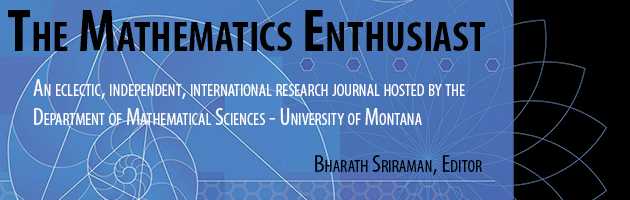•
•12

1-3

#### Abstract

Probability is a difficult topic to teach, not least because it is rather unclear what it actually means. Modern risk communication has tackled general public incomprehension of probability statements by using the metaphor of ‘expected frequencies’ – for example, “of 100 people like you, we would expect 10 to have a heart attack or stroke in the next 10 years.” We show how these ideas can be taken into the classroom as the basis for teaching probability, using frequency tree diagrams as the fundamental representation. Empirical frequency trees can be used to summarise a series of classroom experiments, and then expected frequency trees naturally provide a basis for deriving the rules of probability, and make complex conditional probability calculations reasonably straightforward.

4

10

COinS